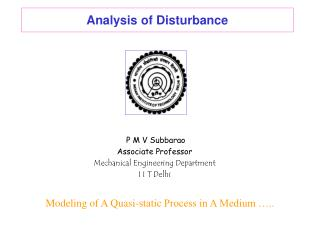DownloadDownload PresentationAnalysis of Disturbance

# Analysis of Disturbance

Télécharger la présentation## Analysis of Disturbance

- - - - - - - - - - - - - - - - - - - - - - - - - - - E N D - - - - - - - - - - - - - - - - - - - - - - - - - - -
##### Presentation Transcript

1. Analysis of Disturbance P M V Subbarao Associate Professor Mechanical Engineering Department I I T Delhi Modeling of A Quasi-static Process in A Medium …..

2. Conservation Laws for a Blissful Fluid

3. Conservation Laws Applied to 1 D Steady disturbance Conservation of Mass: Conservation of Mass for 1DSF: c-u p, r ... C P+dp, r+dr ... Change is final -initial

4. For infinitesimally small disturbance Assume ideal gas conditions for Conservation of Momentum : For steady flow momentum equation for CV: For steady 1-D flow :

5. For infinitesimally small disturbance

6. Nature of Substance • The expressions for speed of sound can be used to prove that speed of sound is a property of a substance. • Using the momentum analysis : • If it is possible to obtain a relation between p and r, then c can be expressed as a state variable. • This is called as equation of state, which depends on nature of substance.

7. Steady disturbance in A Medium c-u p, r ... C P+dp, r+dr ...

8. Speed of sound in ideal and perfect gases • The speed of sound can be obtained easily for the equation of state for an ideal gas because of a simple mathematical expression. • The pressure for an ideal gas can be expressed as a simple function of density and a function molecular structure or ratio of specific heats, gnamely

9. Speed of Sound in A Real Gas • The ideal gas model can be improved by introducing the compressibility factor. • The compressibility factor represents the deviation from the ideal gas. • Thus, a real gas equation can be expressed in many cases as

10. Compressibility Chart

11. Isentropic Relation for A Real Gas Gibbs Equation for a general change of state of a substance: Isentropic change of state:

12. Pfaffian Analysis of Enthalpy For a pure substance : For a change of state: Enthalpy will be a property of a substance iff

13. The definition of pressure specific heat for a pure substance is Gibbs Function for constant pressure process :

14. Gibbs Function for constant temperature process : Divide all terms by dp at constant temperature:

15. Isentropic Relation for A Real Gas

16. Speed of sound in real gas

17. Speed of Sound in Almost Incompressible Liquid • Even flowing Liquid normally is assumed to be incompressible in reality has a small and important compressible aspect. • The ratio of the change in the fractional volume to pressure or compression is referred to as the bulk modulus of the liquid. • For example, the average bulk modulus for water is 2 X109N/m2. • At a depth of about 4,000 meters, the pressure is about 4 X 107N/m2. • The fractional volume change is only about 1.8% even under this pressure nevertheless it is a change. • The compressibility of the substance is the reciprocal of the bulk modulus. • The amount of compression of almost all liquids is seen to be very small.

18. The mathematical definition of bulk modulus as following:

19. Speed of Sound in Solids • The situation with solids is considerably more complicated, with different speeds in different directions, in different kinds of geometries, and differences between transverse and longitudinal waves. • Nevertheless, the speed of sound in solids is larger than in liquids and definitely larger than in gases. • Sound speed for solid is:

20. Speed of Sound in Two Phase Medium • The gas flow in many industrial situations contains other particles. • In actuality, there could be more than one speed of sound for two phase flow. • Indeed there is double chocking phenomenon in two phase flow. • However, for homogeneous and under certain condition a single velocity can be considered. • There can be several models that approached this problem. • For simplicity, it assumed that two materials are homogeneously mixed. • The flow is mostly gas with drops of the other phase (liquid or solid), about equal parts of gas and the liquid phase, and liquid with some bubbles.